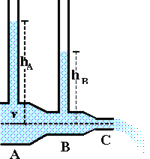# Hydrodynamics/Pressure Problem

## Homework Statement

Water flows through the pipe, as shown in the figure below and exits to the atmosphere at the right end of section C.The diameter of the pipe is 2.04 cm at A, 1.02 cm at B, and 0.850 cm at C. The gauge pressure in the pipe at the center of section A is 1.25 atm and the flow rate is 0.812 L/s. The vertical pipes are open to the air. Find the level (above the flow midline as shown) of the liquid-air interfaces in the two vertical pipes. Assume laminar nonviscous flow.

## Homework Equations

P = P0 + dgh (d= density) (?)

Bernoulli's Equation: P + 1/2pv^2 = constant

A1V1 = A2V2

## The Attempt at a Solution

First, I converted L/s to m/s. I go the velocity at the beginning of the pipe to be 2.48 m/s. Then I plugged it into the equation A1V1=A2V2=, or rather the fact that the diameter decreases by half means the velocity is quadrupled (since area is the square of the radius). Then plugging into the Bernoulli's Equation, i foudn the atmospheric pressure at the second vertical tunnel to be 12.04 atm. Then I put the values into the first equation to determine height of the fluid. That did not give me the answer, not to mention the values seemed far too high. Also, where does the diameter of C fit in? Please help! Thank you.

$$U= .5\rho v^2 + \rho gz + P$$